5.6. Attractor Solutions in Inflation

For simplicity, let us consider a particularly simple potential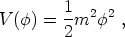(203)

where m has dimensions of mass. We shall also assume initial conditions that at the end of the quantum epoch, which we label as t = 0,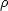~ Mp4.

The slow-roll conditions give that, at t = 0,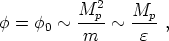(204)

where we have definedm / Mp << 1. We also have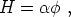(205)

where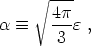(206)

so that the scalar field equation of motion becomes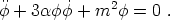(207)

This is solved by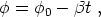(208)

where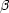m2 / 3. Now, the slow-roll conditions remain satisfied provided that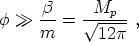(209)

and therefore, (208) is valid for a time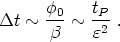(210)

Why is this important? This is important because (208) is an attractor solution! Let's see how this arises. Consider perturbing (208) by writing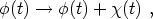(211)

where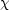(t) is a "small" perturbation, substituting in to the equation of motion and linearizing in. One obtains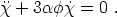(212)

This equation exhibits two solutions. The first is just a constant, which just serves to move along the trajectory. The second solution is a decaying mode with time constant tc = (3)-1. Since tc <<t, all solutions rapidly decay to (208) - it is an attractor.